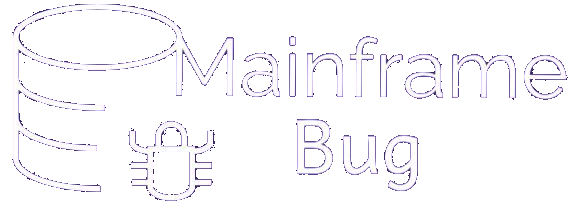## VSAM Quiz

### VSAM Quiz 1-10

• Q1 - Which of the following VSAM clusters support only fixed length records?
• A - RRDS
• B - LDS
• C - KSDS
• D - ESDS
• Answer : A - RRDS
• Q2 - For which of the following VSAM cluser we can specify FREESPACE
• A - RRDS
• B - LDS
• C - KSDS
• D - ESDS
• Answer : C - KSDS
• Q3 - In which type of VSAM cluster records added at the end of the data set?
• A - RRDS
• B - LDS
• C - KSDS
• D - ESDS
• Answer : D - ESDS
• Q4 - The types of processing supported by RRDS are
• A - sequential
• B - direct
• C - skip-sequential
• D - All of above
• Answer : D - All of above
• Q5 - Spanned records can be found in
• A - ESDS
• B - KSDS
• C - RRDS
• D - option A or B
• E - option A or C
• Answer : D - option A or B
• Q6 - If the key of a KSDS record begins in the second field (first field is 25 bytes long), the offset in the KEYS parameter is
• A - 24
• B - 25
• C - 26
• D - None of above
• Answer : B - 25
• Q7 - Alternate Index is a special type of
• A - ESDS
• B - KSDS
• C - RRDS
• D - None of above
• Answer : B - KSDS
• Q8 - Specifying CISZ(4096) at the cluster level for a KSDS results in
• A - Data and index CISZ 4k each
• B - VSAM ignoring the specification
• C - Index CISZ 4K; data CISZ selected by VSAM
• D - Data CISZ 4k; index CISZ selected by VSAM
• Answer : D - Data CISZ 4k; index CISZ selected by VSAM
• Q9 - When defining a KSDS, which of the following parameters is NOT required?
• A - VOLUMES
• B - KEYS
• C - NAME
• D - Space allocation (either CYL, REC or TRK)
• Answer : A - VOLUMES
• Q10 - Default RECORD SIZE for a NONSPANNED data set is
• A - (4086 32600)
• B - (80 80)
• C - (4089 4089)
• D - (100 32600)
• Answer : C - (4089 4089)